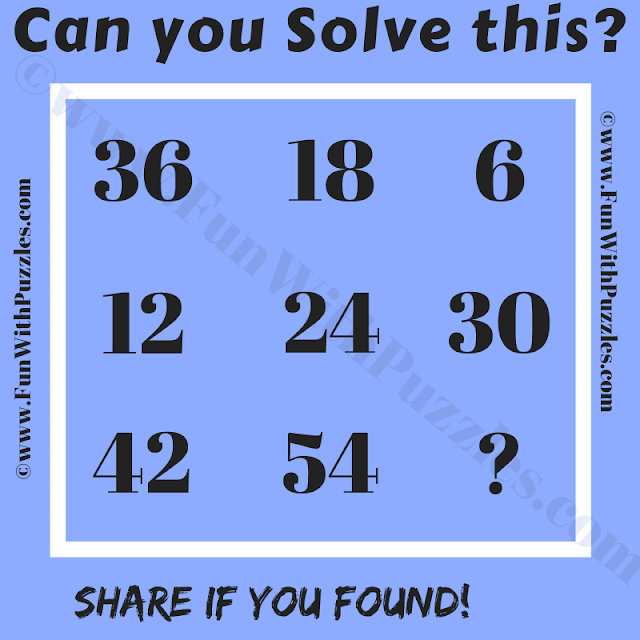This Logic Puzzle is for school-going students. It is definitely going to stump your brain. I created this puzzle around a year back. However, when I want to post this puzzle, I struggled initially to find the answer to this logical puzzle. Sometimes the hidden logic is easy and our mind starts thinking complex logical and mathematical permutations and combinations. This is one such simple logical reasoning puzzle. Study the given numbers in this puzzle picture carefully and find the value of the missing number which will replace the question mark.
This is an easy puzzle and most of the readers here should be able to crack this puzzle quite easily. Write down your answer along with the logical reasoning used to solve this puzzle in the comments box.Can you solve this Logic Puzzle?

The answer to this "Logical Reasoning Puzzle", can be viewed by clicking on the answer button.